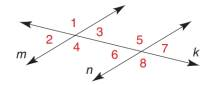Chapter 2.1, Problem 18EElementary Geometry For College St...

7th Edition
Alexander + 2 others
ISBN: 9781337614085

Solutions

Chapter
SectionElementary Geometry For College St...

7th Edition
Alexander + 2 others
ISBN: 9781337614085
Textbook Problem

Given: m ∥ n Transversal k m ∠ 1 = 5 x + y m ∠ 2 = 3 x + y m ∠ 8 = 3 x + 5 y Find: x , y , and m ∠ 8Exercise 1 7 - 1 9

To determine

To find:

The values of x, y and the measure of the given angle.

Explanation

If there exists two parallel lines cut by a transversal then the pair of exterior angles on the same side of transversal is supplementary.

The sum of angles that falls on the straight line is 180°.

Given:

The given figure is,

mn cut by a transversal k.

m1=5x+y, m2=3x+y and m8=3x+5y.

The given angle is 8.

Calculation:

Consider the given figure,

From the figure, mn with transversal k.

1 and 2 fall on the straight line m.

m1+m2=1805x+y+3x+y=1808x+2y=1804x+y=90.......(1)

From the figure (1), mn with transversal k.

4 and 6 are the exterior angles on the same side of transversal k

Still sussing out bartleby?

Check out a sample textbook solution.

See a sample solution

The Solution to Your Study Problems

Bartleby provides explanations to thousands of textbook problems written by our experts, many with advanced degrees!

Get Started

Evaluate the iterated integral: 0401ycosxdydx

Calculus: Early Transcendental Functions

Expand each expression in Exercises 122. (3x+1)(2x2x+1)

Finite Mathematics and Applied Calculus (MindTap Course List)

For , f′(x) =

Study Guide for Stewart's Multivariable Calculus, 8th

In Exercises 110, evaluate the expression. P(24,8)

Finite Mathematics for the Managerial, Life, and Social Sciences

Solve each equation. x58x4+25x338x2+28x18=0

College Algebra (MindTap Course List)